## Symon.AI help center

### Formula

Abstract

Use the Formula tool to apply a formula to an existing column. The tool will create a new column with the transformed data.

Add a new column by applying formula(s) to an existing column. The tool creates a new column with the transformed data.

With the Output column(s) option, you can add new columns or replace existing selected columns in your data set. The columns append to your data set, using the formula name as the column name. This option is available in both the standard pipe and the big pipe.

You can click the Info icon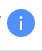in the to-do sidebar to see a list of all formula definitions with examples.

#### Formula functions

Combine formulas to transform data into an existing column. Symon.AI creates a new column with the output.

Table 27. Formula functions

Function

Description

Example

Syntax

Notes

Abs

Returns the absolute value of a number.

If you want to show the difference between this month's sales and last month's sales as a percentage, but you do not want the percent change to be a negative value, use a formula similar to the following example:

ABS(Current - Prior)/Prior

In this example, the formula is subtracting last month's sales from this month's sales and dividing it by last month's sales to get the percentage difference.

Abs(Number)

• Number: The number of which you want the absolute value.

Acos

Returns the arccosine of a number.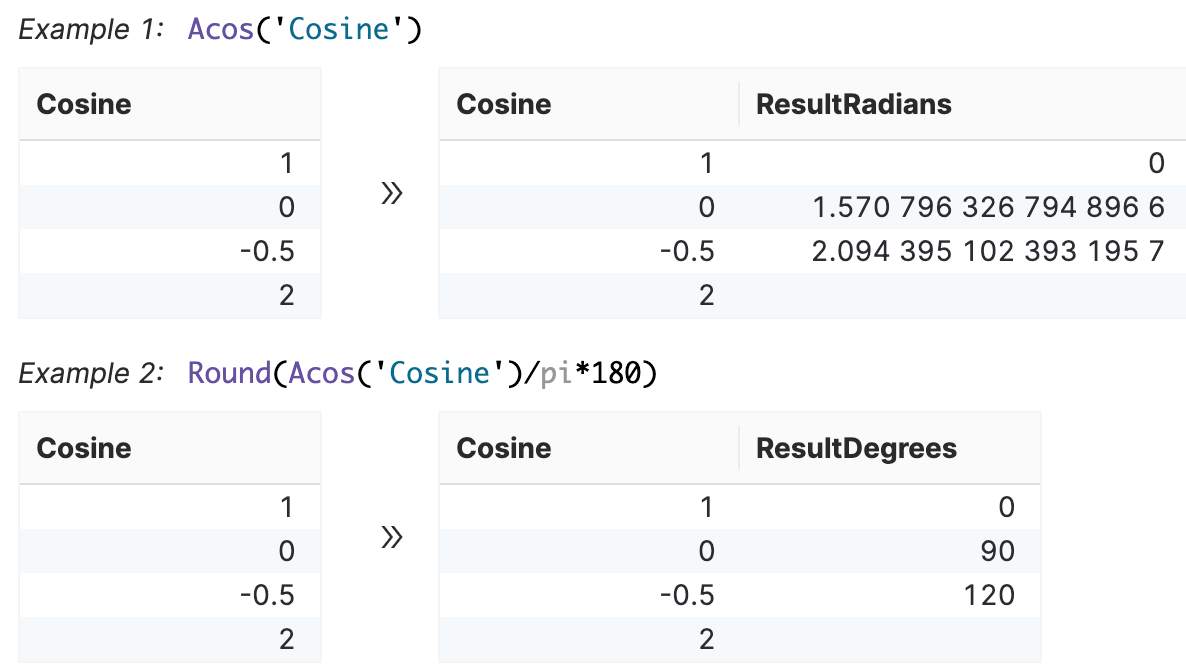Acos(Number)

• Number: The cosine of the acosine angle you want, must be from -1 to 1.

Asin

Returns the arcsine of a number.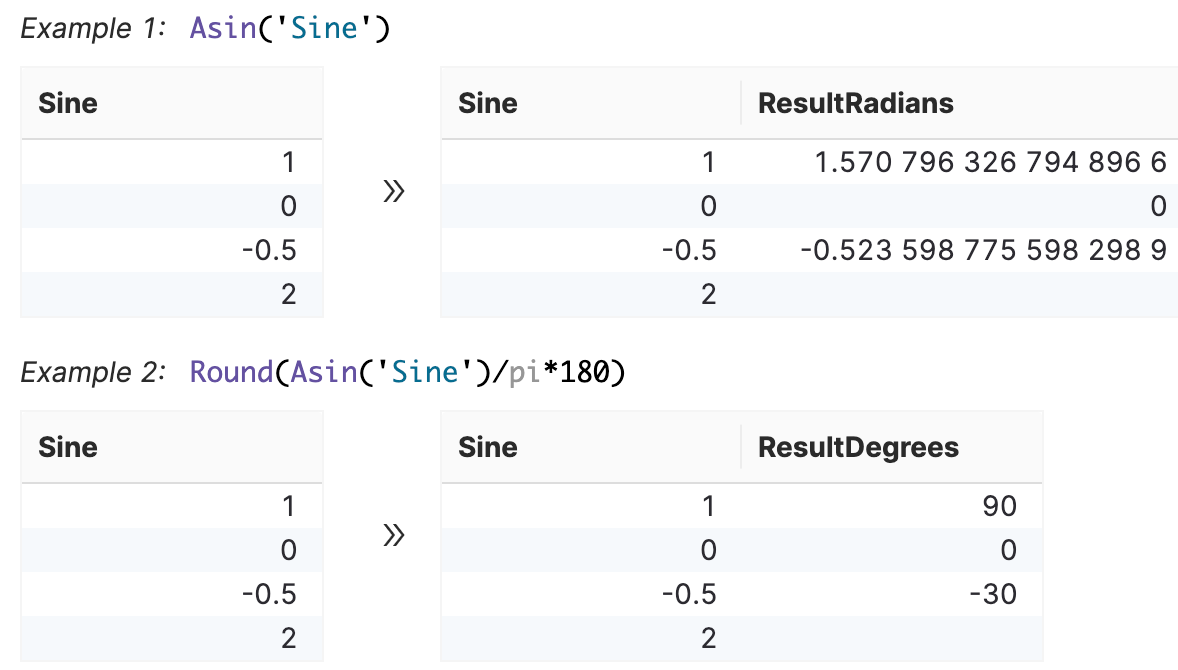Asin(Number)

• Number: The sine of the asine angle you want, must be from -1 to 1.

Atan

Returns the arctangent of a number.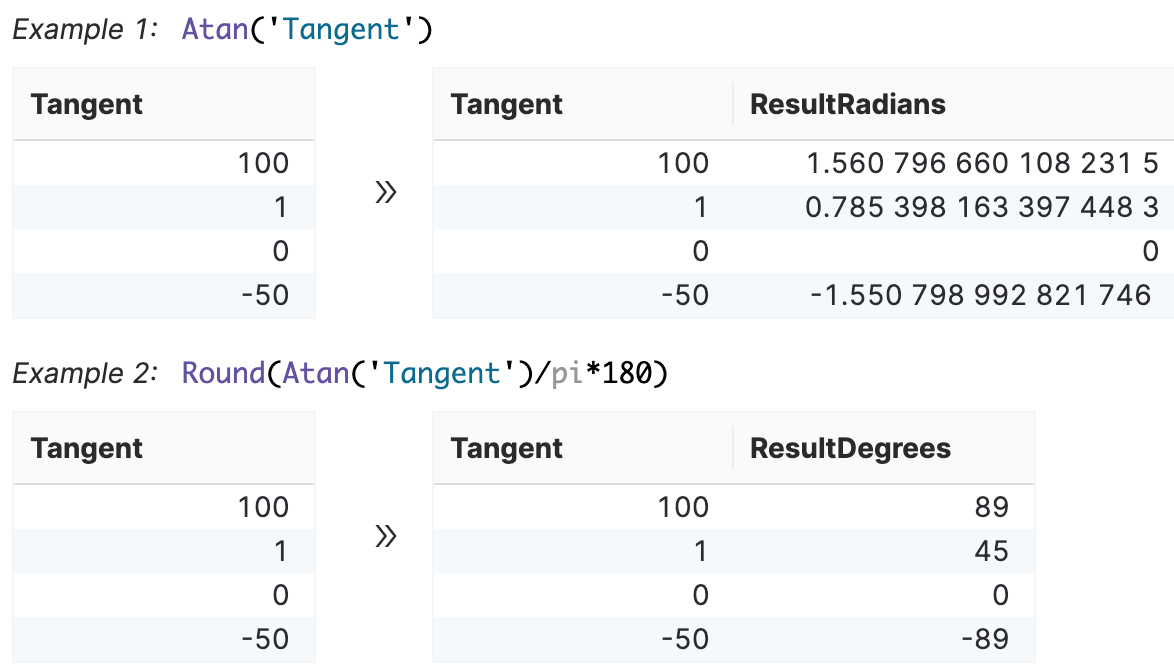Atan(Number)

• Number: The tangent of the angle you want.

Ceil

Rounds a number up to the nearest integer.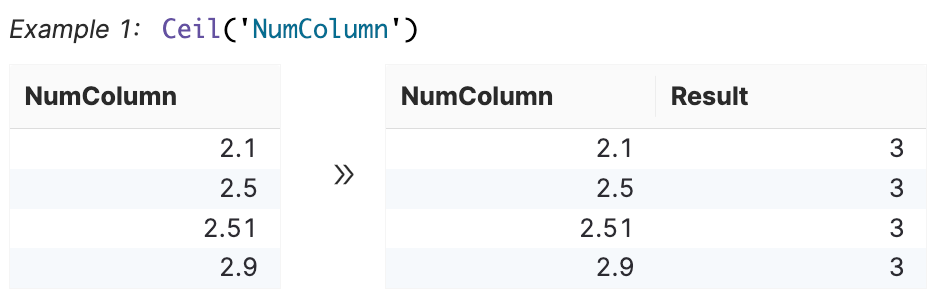Ceil(Number)

• Number: The number or column that you want to round.

Concat

Concatenates all the given text and numbers.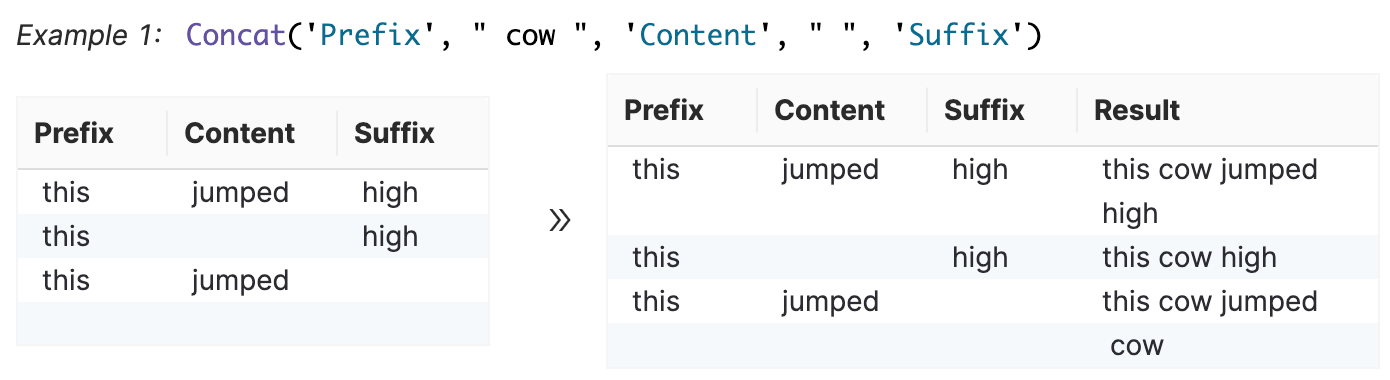Concat(Texts/Numbers...)

• Texts/Numbers...: Any number of columns (Text or Number), user input text or numbers to concatenate with each other.

Contains

Returns either true or false if Text contains the specified Target text.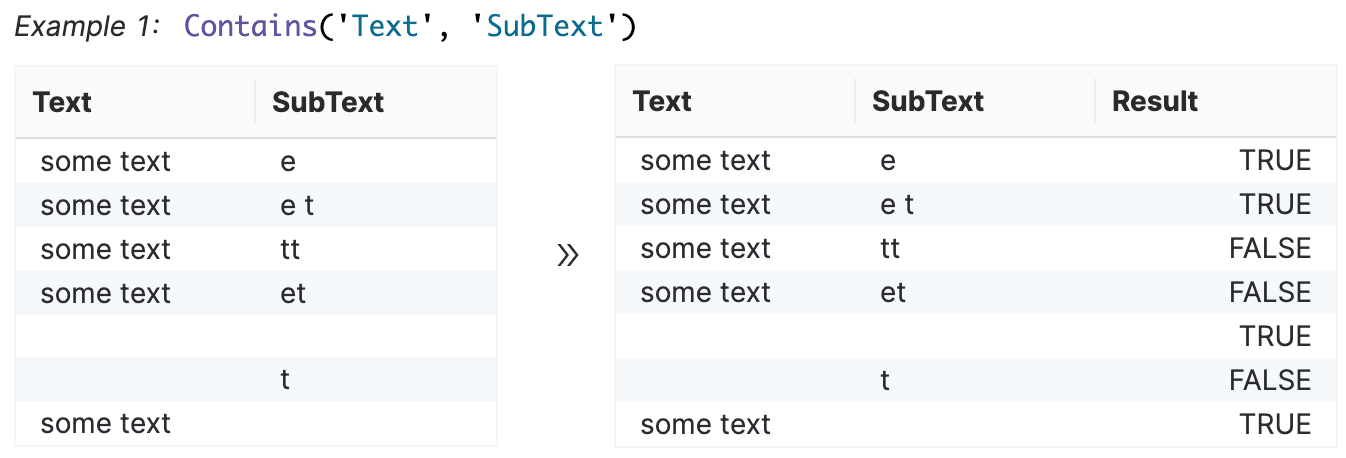Contains(Text,Contained text)

• Text: The text containing the text you want to find. This can be a column or user input text.

• Contained text: The text you want to find. This can be a column or user input text.

Cos

Returns the cosine of a given angle.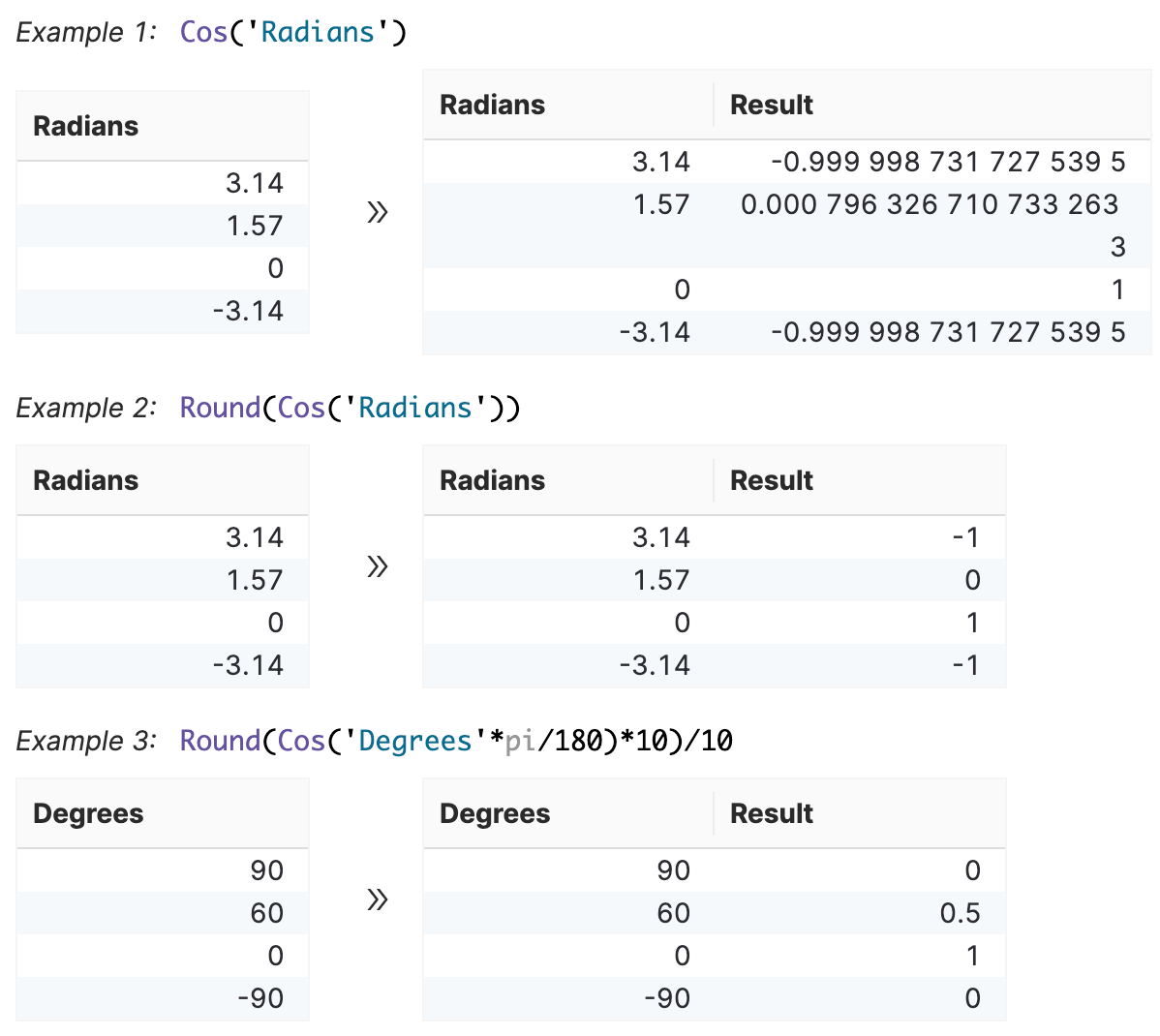Cos(Number)

• Number: The angle in radians for which you want the cosine.

Date

Returns a date given the specified Year, Month, and Day.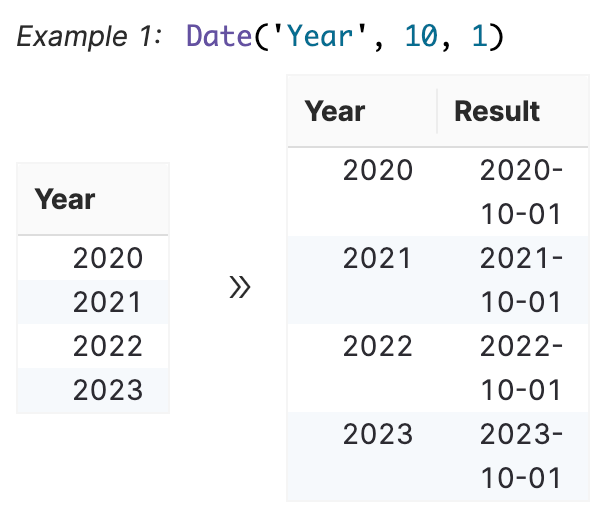Date(Year,Month,Day)

• Year: A four digit number representing the year or column.

• Month: A one or two digit number representing the month or column.

• Day: A one or two digit number representing the day of the month or column.

Endswith

Returns either true or false if Text ends with the specified Ending text.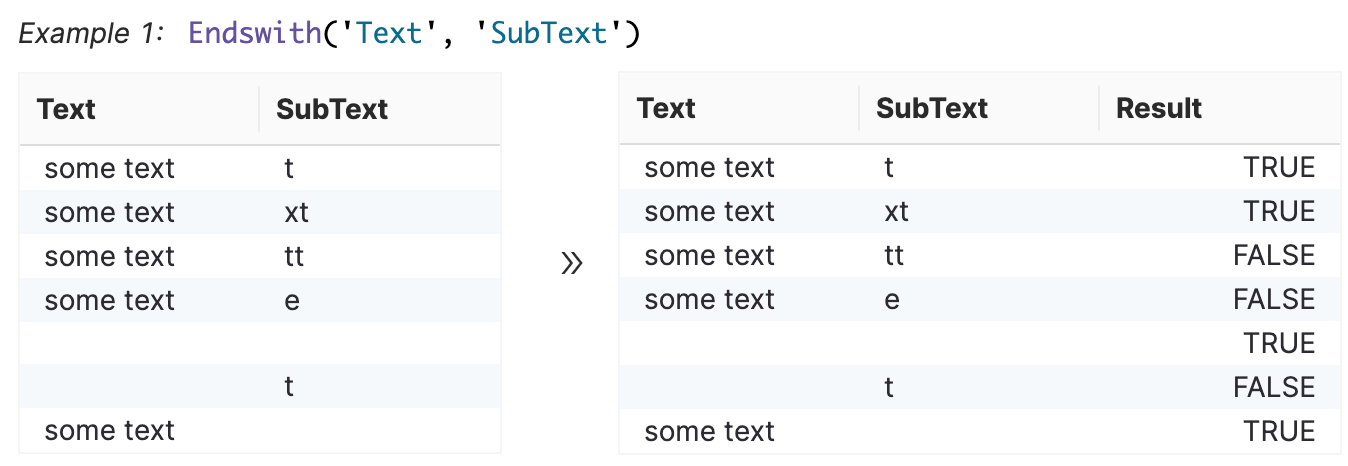Endswith(Text,Ending text)

• Text: The text containing the text you want to find. This can be a column or user input text.

• Ending text: The text you want to find. This can be a column or user input text.

Floor

Rounds a number down to the nearest integer.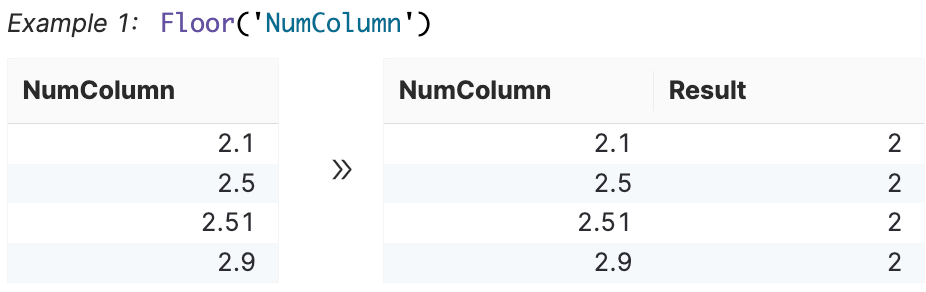Floor(Number)

• Number: The number or column that you want to round.

If

Returns the first input if true and the second input if false.

If the sale amount is greater than $100, you receive a 5% commission. Otherwise, you receive a 2% commission. IF(Data.Value > 100, Data.Value * 0.05, Data.Value * 0.02) Nested IF() example: If your sale amount is greater than$100, you receive 5% commission. If your sale is greater than $50, you receive 3%; otherwise, you receive 2%: IF(Data.Value > 100, Data.Value * 0.05, IF(Data.Value > 50, Data.Value * 0.03, Data.Value * 0.02)) If(Condition,True Result,False Result) • Condition: The condition you want to test. • True Result: The value to return if condition is true. • False Result: The value to return if condition is false. Isnull Returns true for empty rows. Returns false for records that are not empty.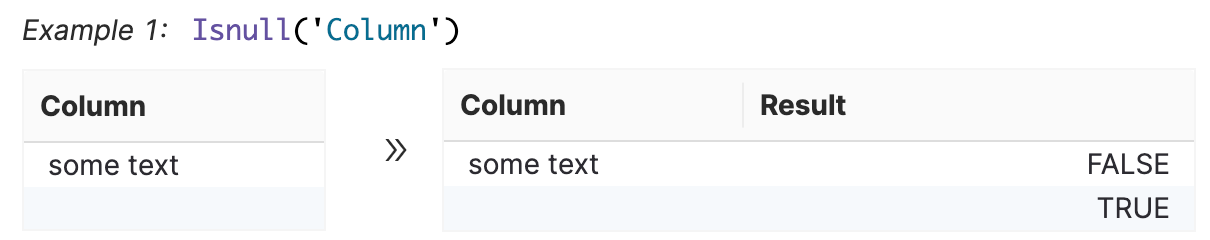Isnull(Column) • Column: The column to check for empty state. Log10 Returns the base- 10 logarithm of a number.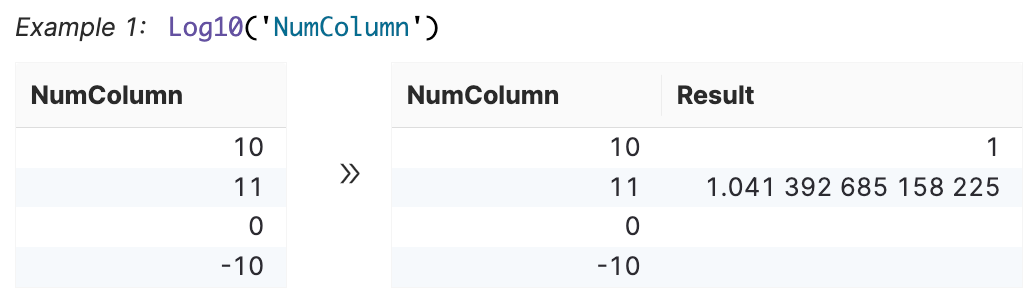Log10(Number) • Number: The positive real number for which you want the base- 10 logarithm. Log2 Returns the base-2 logarithm of a number.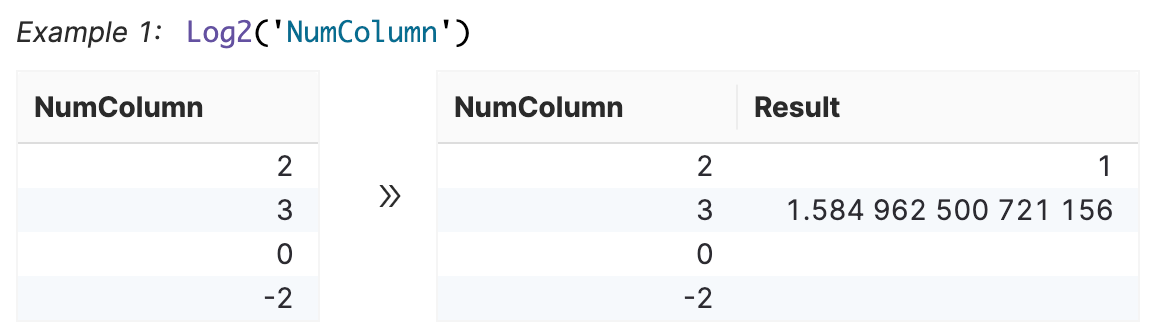Log2(Number) • Number: The positive real number for which you want the base-2 logarithm. Max Returns the maximum value between columns for numbers and dates. If, for each deal, you receive a 5% commission, but there is a minimum of$5 per deal you earn, you can use the following formula to determine whether the 5% commission is higher than the $5 minimum: MAX(Data.Value*0.05,$5)

Max(Numbers/Dates...)

• Numbers/Dates...: Any columns (Number or Date), or user input numbers or dates of which you want to find the maximum.

• If you have an If statement similar to the following example: IF(A>B,A,B), you may want to use the MAX operator.

Min

Returns the minimum value between columns for numbers and dates.

If 5% commission is paid on each deal to a maximum of $100, the following formula pays either 5% commission or$100, whichever is less:

MIN(Data.Value*0.05 , 100)

MIN(Data.Value)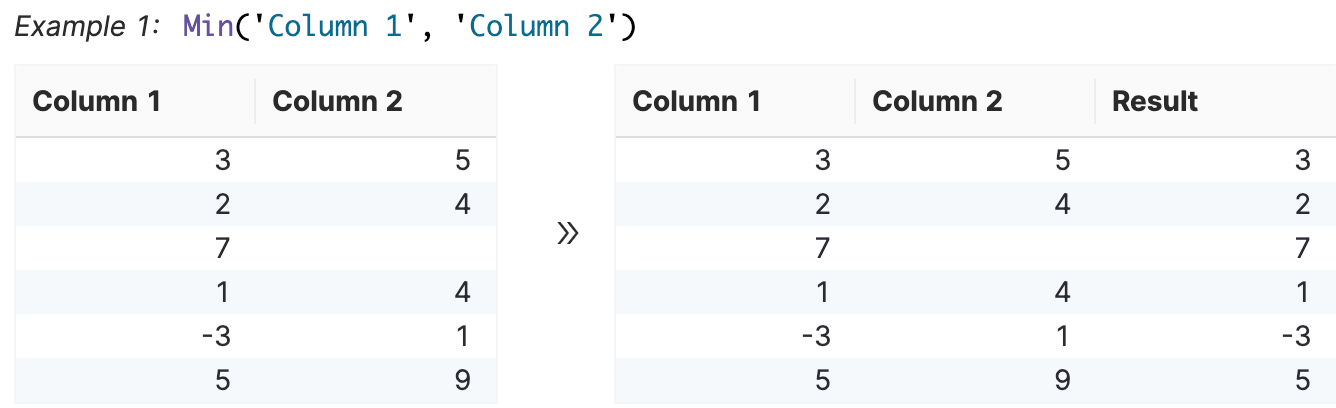Min(Numbers/Dates...)

• Numbers/Dates...: Any columns (Number or Date), or user input numbers or dates of which you want to find the minimum.

• If you have an If statement similar to the following example: IF(A>B,A,B), you may want to use the M operator.

Mround

Returns a number rounded to the nearest multiple.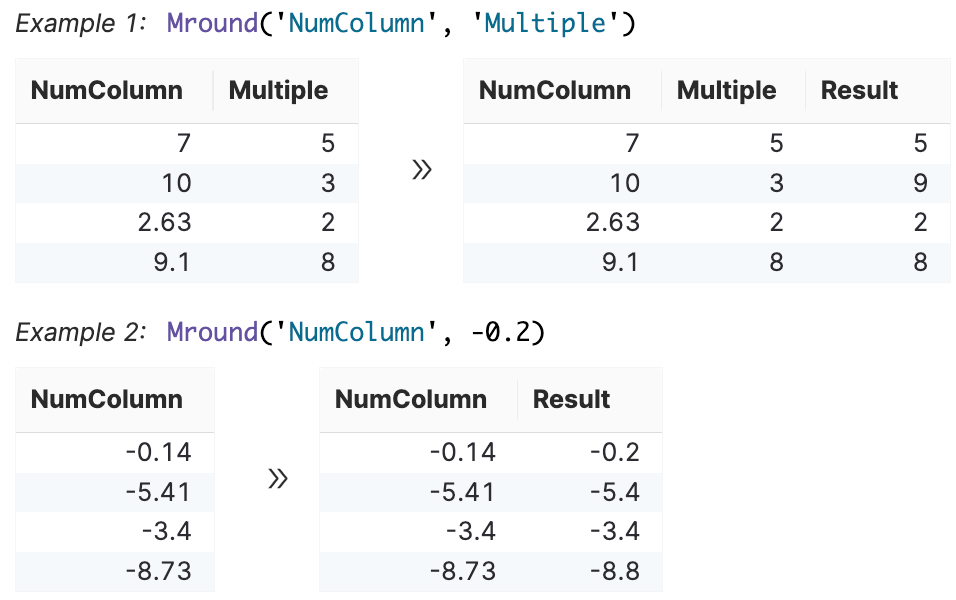Mround(Number,Multiple)

• Number: The value to round, either a numeric value or column.

• Multiple: The multiple used to round the number, either a numeric value or column.

Now

Returns the current time.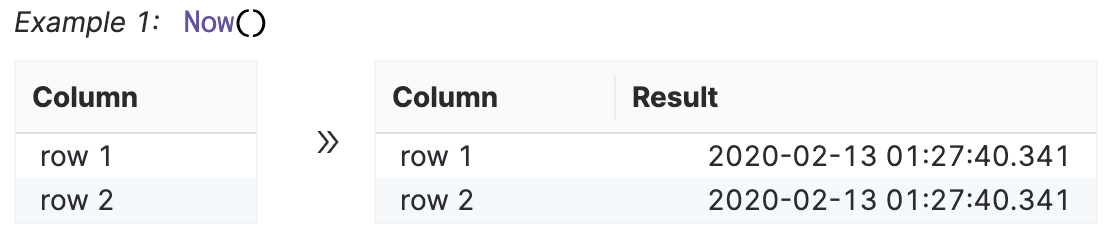Now()

Pad

Adds leading or trailing characters to the text to meet the specified length.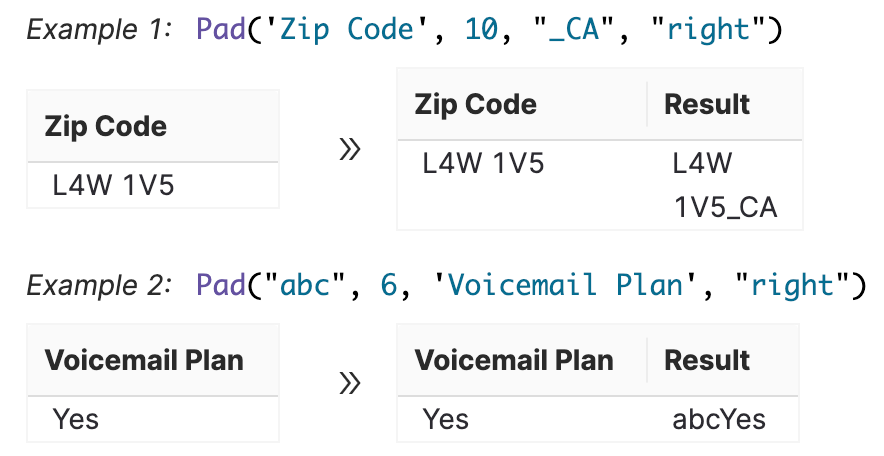Pad(Text,Text length,Pad text,Pad side)

• Text: Column from data or user input.

• Text length: Number of characters or column that indicates the text length for each row.

• Pad text: User input or column, that contains single/multiple characters or whitespace. If left empty, it defaults to white space.

• Pad side: "left" or "right" or a column that indicates the pad direction for each record.

Pow

Returns the result of a number raised to a power.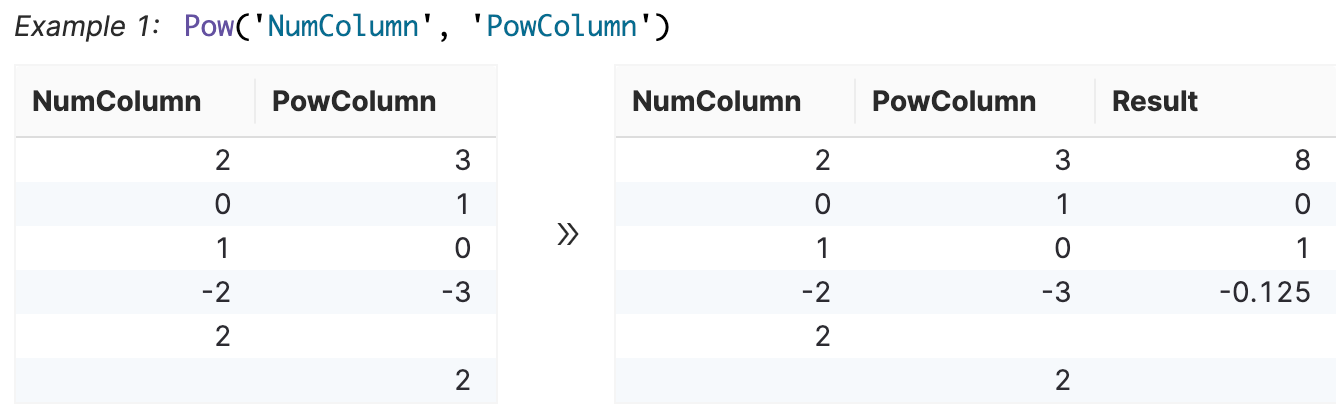Pow(Number,Power)

• Number: The base number.

• Power: The exponent to which the base number is raised.

Round

Rounds a number to a specified number of digits.

If you want to round your results to the nearest cent, use a formula similar to this example:

ROUND(Result, 2)

In this example, the formula rounds the output to 2.

Round(Number,Number of digits)

• Number: The number or column that you want to round.

• Number of digits: The number of digits to which you want to round to.

Sin

Returns the sine of a given angle.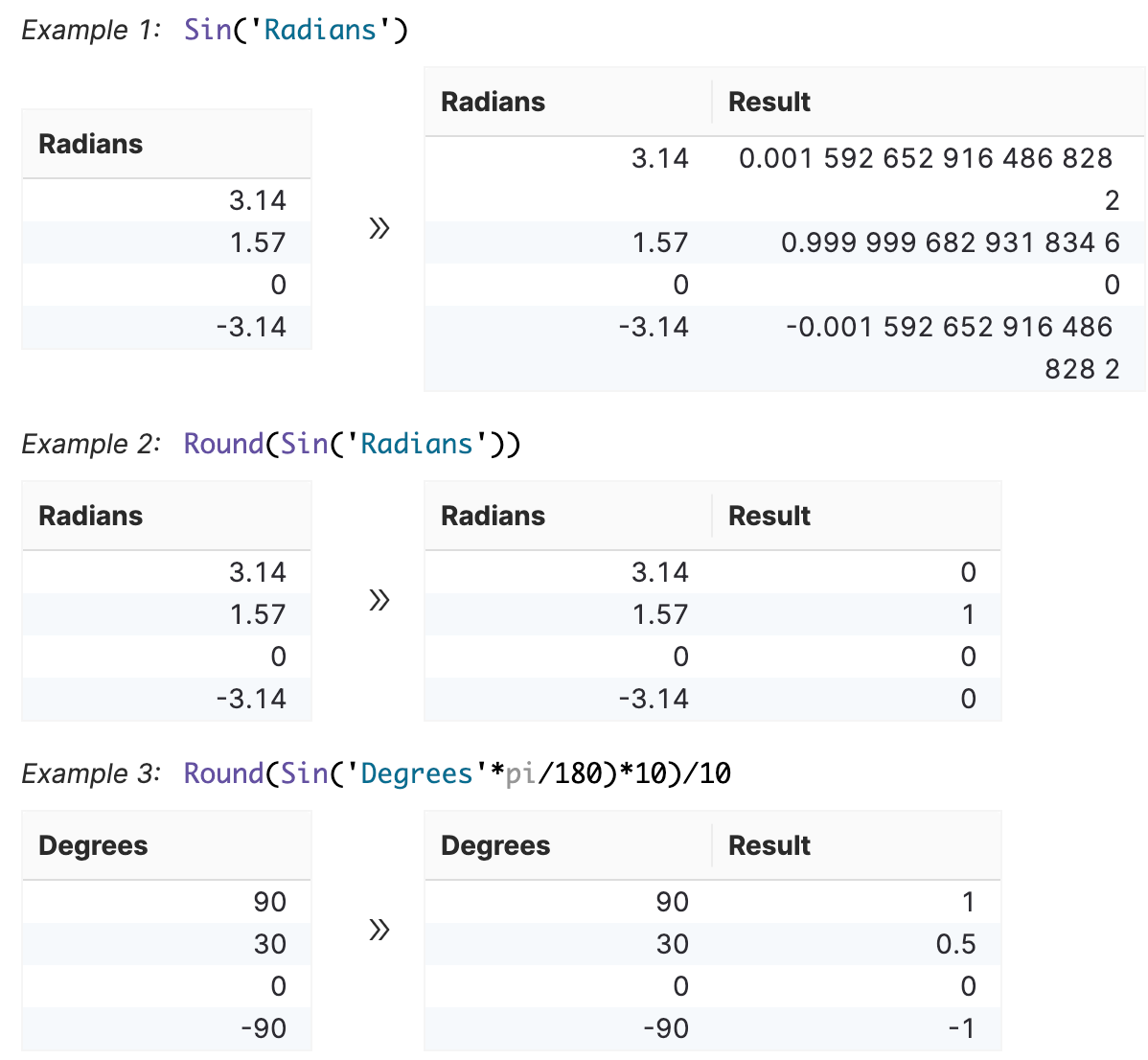Sin(Number)

• Number: The angle in radians for which you want the sine.

Sqrt

Returns a square root of a number.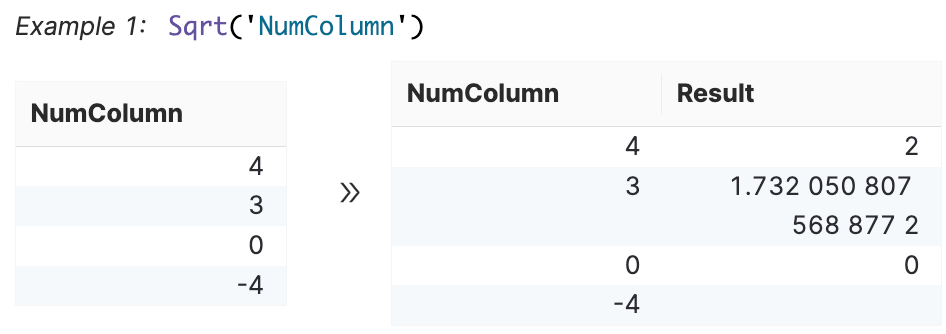Sqrt(Number)

• Number: The number for which you want the square root.

Startswith

Returns either true or false if Text starts with the specified Starting text.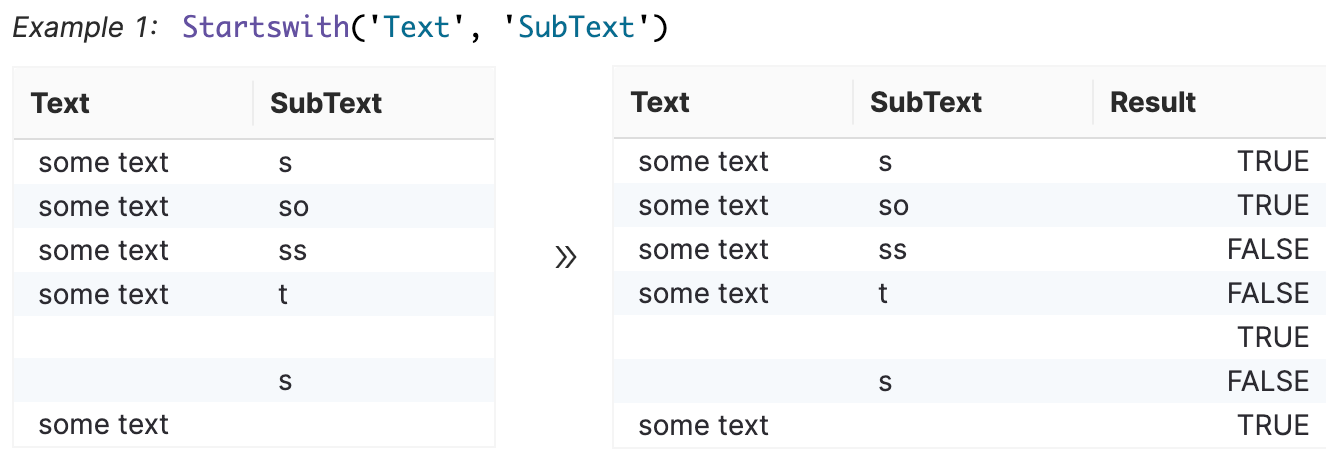Startswith(Text,Starting text)

• Text: The text containing the text you want to find. This can be a column or user input text.

• Starting text: The text you want to find. This can be a column or user input text.

Tan

Returns the tangent of a given angle.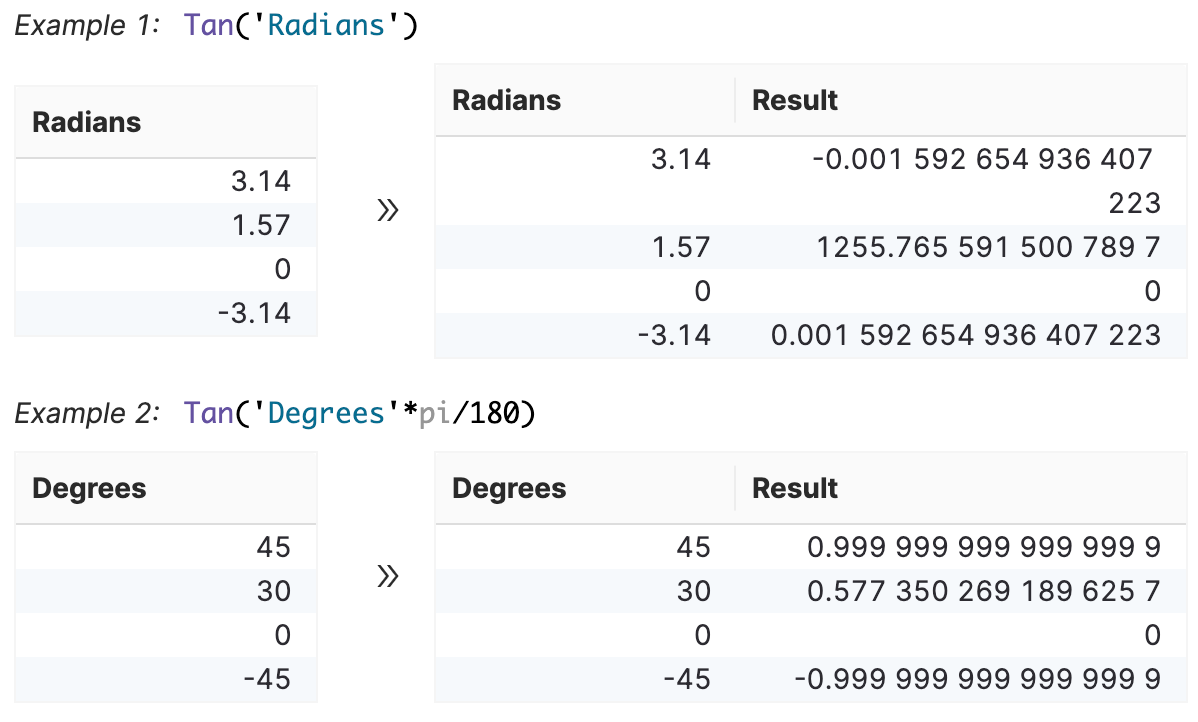Tan(Number)

• Number: The angle in radians for which you want the tangent.

#### Operators

Symon.AI offers the following types of calculation operators to use in your formulas:

Table 28. Operators

Operator

Description

Example

Add +

Returns result of the addition of specified numbers.

formula('col1')+formula('col2').

For example: Ceil('CreditValue')+Ceil('Commission')

and

Filters data based on the specified conditions. Use to combine multiple conditions.

and(value1, value2)

Divide /

Returns the result of the division of specified numbers.

formula('col1')/formula('col2')

For example: Abs('Commission')/Abs('ManualPay')

Equal ==

Returns a true result if the number is equal to another number. If the number is not equal, it returns a false result.

formula('col1')==formula('col2')

For example: Abs('Commission')==Abs('ManualPay')

Less than <

Returns a true result if the left number is less than the right number. If the right number is greater, it will return a false value.

formula('col1')<formula('col2')

For example: Abs('Commission')<Abs('ManualPay')

Greater than >

Returns the result of the number is greater than another number.

formula('col1')>(formula('col2').

For example: Ceil('CreditValue')>Ceil('Commission')

Greater than or equal >=

Returns the result of the number is greater than or equal to another number.

formula('col1')>=(formula('col2').

For example: Ceil('CreditValue')>=Ceil('Commission')

Less than or equal <=

Returns the result of the number less than or equal to another number.

number1<=number2

For example: 30<=45

Multiply *

Returns the result of the multiplication of specificied numbers.

formula('col1')*formula('col2')

For example: Ceil('Rate')*Ceil('CreditValue')

Module %

Returns the remaining value after the number is divided by a divisor.

formula('col1')%formula('col2').

For example: Ceil('CreditValue')&Ceil('Commission')

not

Returns the value when one condition is not true.

not(value1, value2)

Not equal !=

Returns a true result if the number is not equal to another number. If the number has the same value, it returns a false result.

formula('col1')!=formula('col2')

For example: Abs('Commission')!=Abs('ManualPay')

or

Filters data based on the specified conditions. Use to combine multiple conditions. Need at least one condition to be true or both conditions true.

or(value1, [value2])

Subtract -

Returns the result of the the subtraction of specified numbers.

formula('col1')-formula('col2')

For example: Ceil('CreditValue')-Ceil('Commission')

Text ""

A text value within a formula.

"value"

xor

Filters data based on the specified conditions. Use to combine multiple conditions. Need at least one condition to be false or both conditions false.

xor(value1, [value2])

#### Constants

Symon.AI offers the following types of constant operators to use in your formulas:

Table 29. Constants

Constant

Description

Example

Natural base e

Returns the natural logarithm of a value to the base of the mathematical constant e.

=e

phi

Returns the sum of the value of two numbers based on the phi mathematical constant value.

=phi

pi

Returns the sum of the value based on the pi mathematical constant value.

=pi

tau

Returns the sum of the value of two numbers based on the tau mathematical constant value.

=tau

#### Data types

Symon.AI uses the following data types to construct formulas:

• Boolean: Used to indicate the result value of either True or False.

• Double quote "": Used to to create strings or text, such as “This is a string” or “Male”.

• Numeric: Used to indicate the value is in numeric form, either float or integer, such as 1 or 3.12.

• Single quote '': Used to indicate columns, such as ‘Age’ refers to the column Age.

#### Limitations

The behavior of round() for floats can be surprising because most decimal fractions cannot be represented exactly as a float.

For example, round(2.675, 2) will return 2.67 instead of the expected 2.68.

##### Using Row Viewer Format

When you use the Row Viewer format, the format inherits the format from the preceding tool. But, some formulas may have a different output, based on one of the following scenarios:

• There is not a preceding tool to inherit the format from.

• The column types between the two tools are different and cannot inherit the format.

• The function applied in the current tool takes precedence over the inherited format.

Some formula functions affect the decimal place without the Row Viewer format. The following functions always take precedence and prevent the inherited decimal place formatting:

• round()

• floor()

• ceil()

The following functions use the inherited format:

• abs()

• sqrt()

• log10()

• sin()

• cos()

• asin()

• acos()

• atan()

• pow()

if() outputs the same format if the true results and the false results have the same format. If they do not have the same format, the input formats are ignored.

#### Error messaging

The Formula tool can find the following errors in your data set:

• Syntax error: An unknown function is occurring, or the function cannot parse due to a special character, or a missing bracket.

• Invalid argument error: The tool detects that the argument is invalid due to a wrong data type.

• Too many arguments error: The tool detects that there are too many arguments.

• Missing arguments error: The tool detects that there are one or more arguments missing from the range of the number of allowed arguments.

• Unsupported data type error: The tool detects an unsupported data type. The Formula tool only accepts number, boolean, string, and date data types.